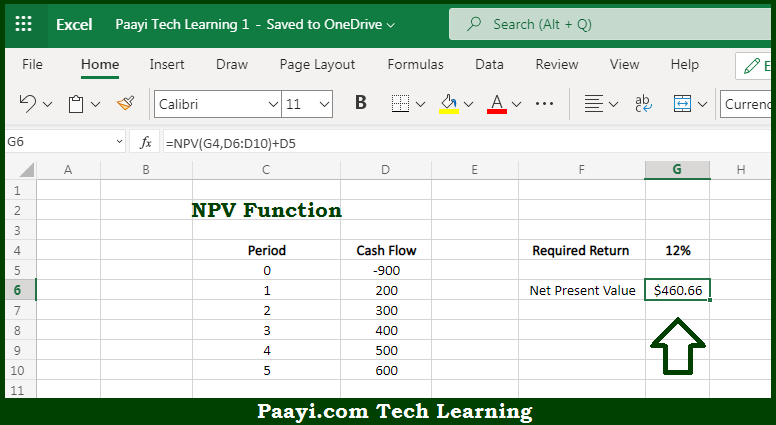# Learn How to Use Microsoft Excel NPV Function

Written by | 0 Comments | 557 Views

In this article, you will learn how to use the Microsoft Excel NPV function and its prime function in Microsoft Excel. You will also get to know the Microsoft Excel NPV function return value and syntax with the help of some examples.

Microsoft Excel NPV Function

The main purpose of the Microsoft Excel NPV function is to calculate the net present value. That implies, with the help of NPV function which is a financial function you can able to calculate the net present value, abbreviated as NPV of an investment using a discount rate and a series of future cash flows. So, with the help of the NPV function, you can able to calculate the net present value.

Return Value of NPV Function

The return value will be the net present value.

Syntax of NPV Function

=NPV(rate,[value1], [value2])

Where the arguments:

• rate: This is the discount rate over one period.
• value1: This is the first value(s) that represents the cash flow.
• value2: This is the second value(s) that represents the cash flow (optional).

## How to Use Microsoft Excel NPV Function?So we know that Microsoft Excel NPV function you can able to calculate the net present value. That implies, with the help of NPV function which is the financial function you can able to calculate the net present value, abbreviated as NPV of an investment using a discount rate and a series of future cash flows. So, with the help of the NPV function, you can able to calculate the net present value.